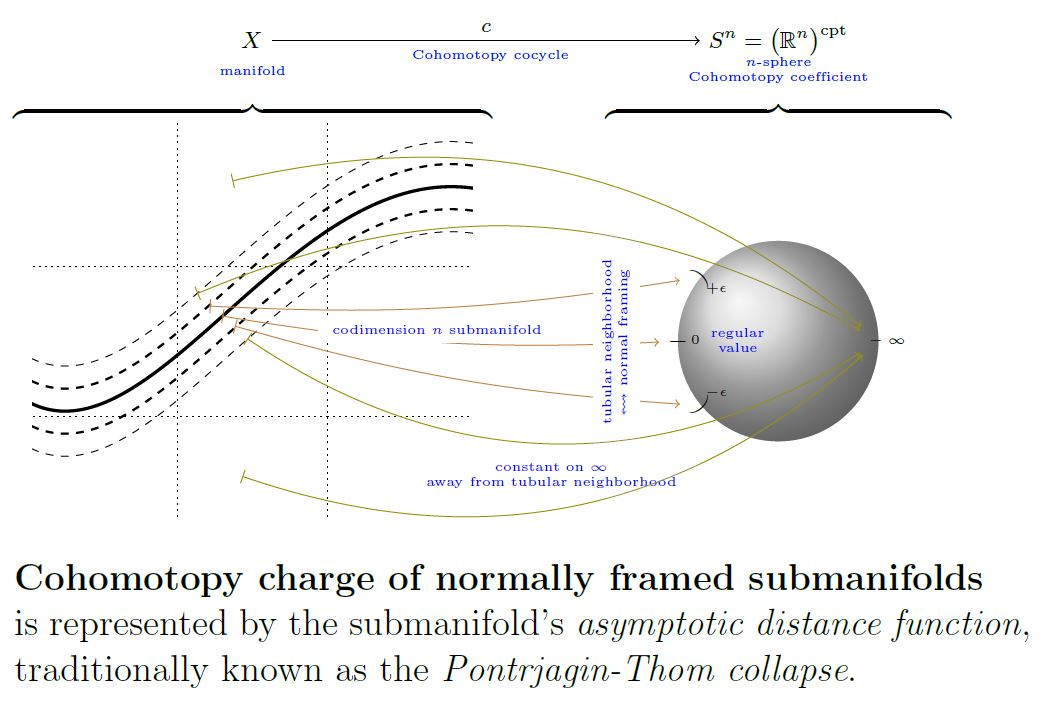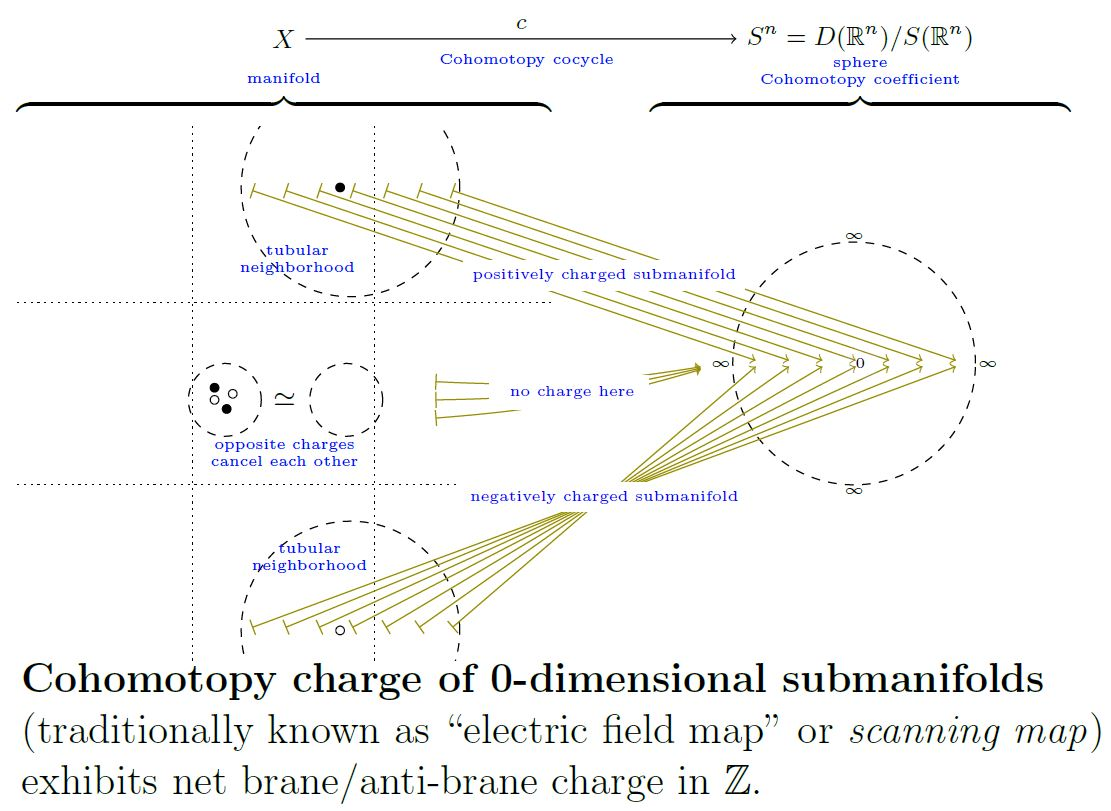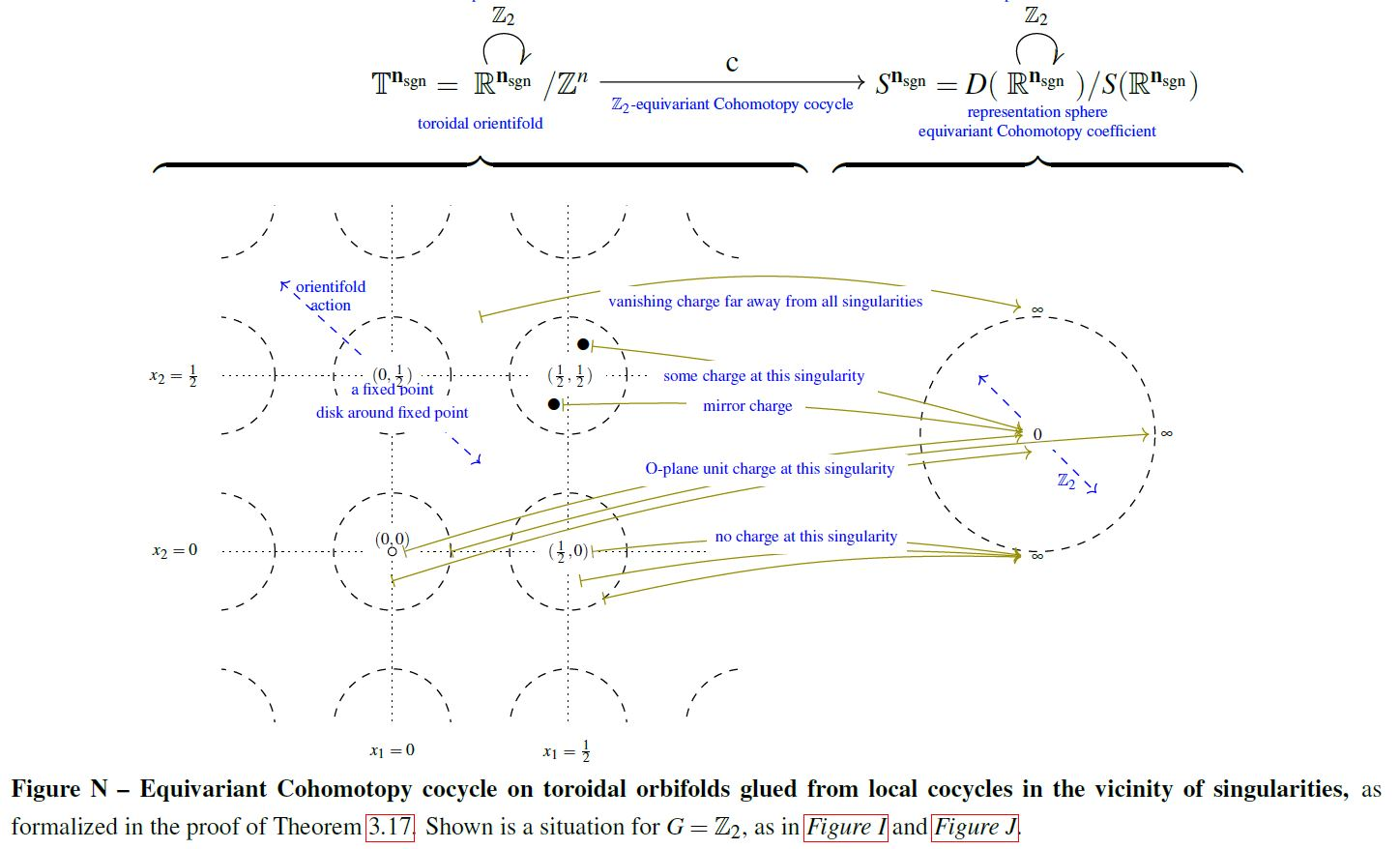Contents

cohomology

# Contents

## Idea

### General

The Cohomotopy charge map is the function that assigns to a configuration of normally framed submanifolds of codimension $n$ their total charge as measured in $n$-Cohomotopy-cohomology theory.

Concretely, this is the function which assigns to a normally framed submanifold its asymptotic normal distance function, namely the distance from the submanifold measured

1. in direction perpendicular to the submanifold, as encoded by the normal framing;

2. asymptotically, regarding all points outside a tubular neighbourhood as being at infinity.graphics grabbed from SS 19

For general $n$ this is known as the “Pontrjagin-Thom collapse construction”.

### For charged points

For maximal codimension $n$ inside an oriented manifold, hence for 0-dimensional submanifolds, hence for configurations of points and with all points regarded as equipped with positive normal framing, the Cohomotopy charge map is alternatively known as the “electric field map” (Salvatore 01 following Segal 73, Section 1, see also Knudsen 18, p. 49) or the “scanning map” (Kallel 98, Manthorpe-Tillmann 13):

In maximal codimension $D \in \mathbb{N}$, the Cohomotopy charge map is thus the continuous function

(1)$Conf\big( \mathbb{R}^D \big) \overset{cc}{\longrightarrow} \mathbf{\pi}^D \Big( \big( \mathbb{R}^D \big)^{cpt} \Big) = Maps^{\ast/\!}\Big( \big(\mathbb{R}^D\big)^{cpt} , S^D\big) = \Omega^{D} S^D$

from the configuration space of points in the Euclidean space $\mathbb{R}^D$ to the $D$-Cohomotopy cocycle space vanishing at infinity on the Euclidean space(which is equivalently the space of pointed maps from the one-point compactification $S^D \simeq \big( \mathbb{R}^D \big)$ to itself, and hence equivalently the $D$-fold iterated based loop space of the D-sphere), which sends a configuration of points in $\mathbb{R}^D$, each regarded as carrying unit charge to their total charge as measured in Cohomotopy-cohomology theory (Segal 73, Section 3).graphics grabbed from SS 19

This has evident generalizations to other manifolds than just Euclidean spaces, to spaces of labeled configurations and to equivariant Cohomotopy. The following graphics illustrates the Cohomotopy charge map on G-space tori for $G = \mathbb{Z}_2$ with values in $\mathbb{Z}_2$-equivariant Cohomotopy:graphics grabbed from SS 19

## Properties

### Characterization of cobordism classes by their Cohomotopy charge

The unstable Pontrjagin-Thom theorem states that Cohomotopy charge faithfully reflects configurations of normally framed submanifolds up to normally framed embedded cobordism, hence that the Pontrjagin-Thom collapse construction induces a bijection between cobordism classes of normally framed submanifolds and the Cohomotopy set in degree the respective codimension:

$\left\{ { { \text{normally framed submanifolds} } \atop { \text{in}\;X\;\text{of codimension}\; n } } \right\} \Big/_{\sim_{cobordism}} \underoverset{\simeq}{cc}{\longrightarrow} \underset{ \mathclap{ \color{blue} { \text{Cohomotopy} \atop \text{set} } } }{ \pi^n\big( X \big) }$

For more details see here.

In good situations this bijection of sets of homotopy classes enhances to a weak equivalence of configuration spaces/cocycle spaces. See Characterization of point configurations by their Cohomotopy charge below.

### Characterization of point configurations by their Cohomotopy charge

In some situations the Cohomotopy charge map is a weak homotopy equivalence and hence exhibits, for all purposes of homotopy theory, the Cohomotopy cocycle space of Cohomotopy charges as an equivalent reflection of the configuration space of points.

#### On Euclidean spaces via plain Cohomotopy

###### Proposition

(group completion on configuration space of points is iterated based loop space)

$Conf \big( \mathbb{R}^D \big) \overset{ cc }{\longrightarrow} \Omega^D S^D$

from the full unordered and unlabeled configuration space (here) of Euclidean space $\mathbb{R}^D$ to the $D$-fold iterated based loop space of the D-sphere, exhibits the group completion (here) of the configuration space monoid

$\Omega B_{{}_{\sqcup}\!} Conf \big( \mathbb{R}^D \big) \overset{ \simeq }{\longrightarrow} \Omega^D S^D$
###### Proposition

(Cohomotopy charge map is weak homotopy equivalence on sphere-labeled configuration space of points)

For $D, k \in \mathbb{N}$ with $k \geq 1$, the Cohomotopy charge map (1)

$Conf \big( \mathbb{R}^D, S^k \big) \underoverset{\simeq}{cc}{\longrightarrow} \Omega^D S^{D + k}$

is a weak homotopy equivalence from the configuration space (here) of unordered points with labels in $S^k$ and vanishing at the base point of the label space to the $D$-fold iterated loop space of the D+k-sphere.

#### On closed manifolds via twisted Cohomotopy

The May-Segal theorem generalizes from Euclidean space to closed smooth manifolds if at the same time one passes from plain Cohomotopy to twisted Cohomotopy, twisted, via the J-homomorphism, by the tangent bundle:

###### Proposition

Let

1. $X^n$ be a smooth closed manifold of dimension $n$;

2. $1 \leq k \in \mathbb{N}$ a positive natural number.

Then the Cohomotopy charge map constitutes a weak homotopy equivalence

$\underset{ \color{blue} { \phantom{a} \atop \text{ J-twisted Cohomotopy space}} }{ Maps_{{}_{/B O(n)}} \Big( X^n \;,\; S^{ \mathbf{n}_{def} + \mathbf{k}_{\mathrm{triv}} } \!\sslash\! O(n) \Big) } \underoverset {\simeq} { \color{blue} \text{Cohomotopy charge map} } {\longleftarrow} \underset{ \mathclap{ \color{blue} { \phantom{a} \atop { \text{configuration space} \atop \text{of points} } } } }{ Conf \big( X^n, S^k \big) }$

between

1. the J-twisted (n+k)-Cohomotopy space of $X^n$, hence the space of sections of the $(n + k)$-spherical fibration over $X$ which is associated via the tangent bundle by the O(n)-action on $S^{n+k} = S(\mathbb{R}^{n} \times \mathbb{R}^{k+1})$

2. the configuration space of points on $X^n$ with labels in $S^k$.

(Bödigheimer 87, Prop. 2, following McDuff 75)

###### Remark

In the special case that the closed manifold $X^n$ in Prop. is parallelizable, hence that its tangent bundle is trivializable, the statement of Prop. reduces to this:

Let

1. $X^n$ be a parallelizable closed manifold of dimension $n$;

2. $1 \leq k \in \mathbb{N}$ a positive natural number.

Then the Cohomotopy charge map constitutes a weak homotopy equivalence

$\underset{ \color{blue} { \phantom{a} \atop \text{ Cohomotopy space}} }{ Maps \Big( X^n \;,\; S^{ n + k } \Big) } \underoverset {\simeq} { \color{blue} \text{Cohomotopy charge map} } {\longleftarrow} \underset{ \mathclap{ \color{blue} { \phantom{a} \atop { \text{configuration space} \atop \text{of points} } } } }{ Conf \big( X^n, S^k \big) }$

between

1. $(n+k)$-Cohomotopy space of $X^n$, hence the space of maps from $X$ to the (n+k)-sphere

2. the configuration space of points on $X^n$ with labels in $S^k$.

### Pontrjagin-Thom construction

#### Pontrjagin’s construction

##### General

The Pontryagin theorem, i.e. the unstable and framed version of the Pontrjagin-Thom construction, identifying cobordism classes of normally framed submanifolds with their Cohomotopy charge in unstable Borsuk-Spanier Cohomotopy sets, is due to:

(both available in English translation in Gamkrelidze 86),

as presented more comprehensively in:

The Pontrjagin theorem must have been known to Pontrjagin at least by 1936, when he announced the computation of the second stem of homotopy groups of spheres:

• Lev Pontrjagin, Sur les transformations des sphères en sphères (pdf) in: Comptes Rendus du Congrès International des Mathématiques – Oslo 1936 (pdf)

Review:

Discussion of the early history:

##### Twisted/equivariant generalizations

The (fairly straightforward) generalization of the Pontrjagin theorem to the twisted Pontrjagin theorem, identifying twisted Cohomotopy with cobordism classes of normally twisted-framed submanifolds, is made explicit in:

A general equivariant Pontrjagin theorem – relating equivariant Cohomotopy to normal equivariant framed submanifolds – remains elusive, but on free G-manifolds it is again straightforward (and reduces to the twisted Pontrjagin theorem on the quotient space), made explicit in:

• James Cruickshank, Thm. 5.0.6, Cor. 6.0.13 in: Twisted Cobordism and its Relationship to Equivariant Homotopy Theory, 1999 (pdf, pdf)
##### In negative codimension

In negative codimension, the Cohomotopy charge map from the Pontrjagin theorem gives the May-Segal theorem, now identifying Cohomotopy cocycle spaces with configuration spaces of points:

• Peter May, The geometry of iterated loop spaces, Springer 1972 (pdf)

• Graeme Segal, Configuration-spaces and iterated loop-spaces, Invent. Math. 21 (1973), 213–221. MR 0331377 (pdf)

c Generalization of these constructions and results is due to

• Dusa McDuff, Configuration spaces of positive and negative particles, Topology Volume 14, Issue 1, March 1975, Pages 91-107 (doi:10.1016/0040-9383(75)90038-5)

• Carl-Friedrich Bödigheimer, Stable splittings of mapping spaces, Algebraic topology. Springer 1987. 174-187 (pdf, pdf)

#### Thom’s construction

Thom's theorem i.e. the unstable and oriented version of the Pontrjagin-Thom construction, identifying cobordism classes of normally oriented submanifolds with homotopy classes of maps to the universal special orthogonal Thom space $M SO(n)$, is due to:

Textbook accounts:

#### Lashof’s construction

The joint generalization of Pontryagin 38a, 55 (framing structure) and Thom 54 (orientation structure) to any family of tangential structures (“(B,f)-structure”) is first made explicit in

and the general statement that has come to be known as the Pontryagin-Thom isomorphism (identifying the stable cobordism classes of normally (B,f)-structured submanifolds with homotopy classes of maps to the Thom spectrum Mf) is really due to Lashof 63, Theorem C.

Textbook accounts:

Lecture notes:

• John Francis, Topology of manifolds course notes (2010) (web), Lecture 3: Thom’s theorem (pdf), Lecture 4 Transversality (notes by I. Bobkova) (pdf)

• Cary Malkiewich, Section 3 of: Unoriented cobordism and $M O$, 2011 (pdf)

• Tom Weston, Part I of An introduction to cobordism theory (pdf)